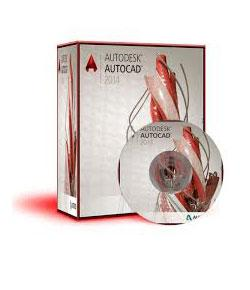# Introduction To AutoCAD

17 Questions | Total Attempts: 4787SettingsThis is a quick quiz to check the level of underpinning knowledge, based on the Basic Autocad software.

Questions and Answers
• 1.
To draw a line what information must be known? Multiple answers are allowed.
• A.

Starting point

• B.

Height

• C.

Colour

• D.

Length

• E.

Direction

• 2.
To select the centre of a line what option must be selected?
• A.

Ortho

• B.

Dynamic input

• C.

Object snap

• 3.
To draw a horizontal line from right to left what direction would it be?
• A.

90

• B.

180

• C.

0

• D.

270

• 4.
What tool  is being pointed to in the image?
• A.

Break

• B.

Mirror

• C.

Offset

• D.

Move

• 5.
When selecting with the green marquee does the object need to be touched by the green area or be contained to be selected ?
• A.

Contained

• B.

Touching

• 6.
What tool  is being pointed to in the image?
• A.

Copy

• B.

Scale

• C.

Trim

• D.

Offset

• E.

Extened

• 7.
Autocad drawings have what extension?
• A.

.dwt

• B.

.dwg

• C.

.doc

• D.

.jpg

• 8.
What is a block in Autocad?
• A.

A square drawn by four separate lines

• B.

A rectangle drawn with the rectangle tool

• C.

A drawing that can be reused in the current drawing or brought in from another drawing

• 9.
A rectangle drawn with the rectangle tool  is four separate lines or four lines joined together?
• A.

Four lines joined together

• B.

Four seperate lines

• 10.
What tool  is being pointed to in the image?
• A.

Copy

• B.

Offset

• C.

Erase

• D.

Trim

• E.

Scale

• 11.
Does the template set up the machine or does it set up the drawing?
• A.

Machine

• B.

Drawing

• 12.
What is the shortcut key for ortho?
• A.

F5

• B.

Enter

• C.

F10

• D.

F8

• E.

Escape

• 13.
What tool is used to draw a line parallel to a existing line at a exact distance from it?
• A.

Ray

• B.

Ortho

• C.

Offset

• D.

Polyline

• 14.
What type of line is drawn from one to infinity in one direction?
• A.

Poly line

• B.

Ray line

• C.

Construction line

• D.

Dashed Line

• 15.
What tool is used to put a radius on a existing intersection?
• A.

Trim

• B.

Extened

• C.

Ortho

• D.

Fillet

• 16.
L  enter 0,0 @1000<0 @1000<90 @1000<180 @1000<270 What will these commands produce?
• A.

Multiple circles

• B.

A meter by meter square

• C.

Four points randomly placed on the page

• 17.
To plot a drawing is to do what?
• A.

Add a new layer

• B.

Print it

• C.

Add some key points

Related Topics